# Ex.12.2 Q6 Areas Related to Circles Solution - NCERT Maths Class 10

Go back to  'Ex.12.2'

## Question

A chord of a circle of radius $$15\, \rm{cm}$$ subtends an angle of $$60^\circ$$ at the Centre. Find the areas of the corresponding minor and major segments of the circle.

(Use $$\pi ={ }3.14$$ and  $$\sqrt{3}=1.73$$)

## Text Solution

What is known?

A chord of a circle with radius $${(r)} = 15\, \rm{cm}$$ subtends an angle \begin{align}(\theta)=60^{\circ}\end{align} at the centre.

What is unknown?

Area of minor and major segments of the circle.

Reasoning:

In a circle with radius r and angle at the centre with degree measure $$\rm{\theta }$$;

(i) Area of the sector \begin{align} = \frac{{\rm{\theta }}}{360}^{\rm{o}} \times \pi {r^2}\end{align}

(ii) Area of the segment = Area of the sector - Area of the corresponding triangle

Draw a figure to visualize the area to be found out.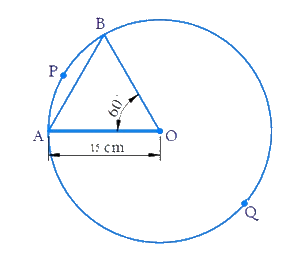Here, \begin{align}\rm{Radius, }r =15\,cm\,\,\,\,\,\,\,\,\,\,{\rm{ \theta }} = {60^{\rm{\circ}}}\end{align}

Visually it's clear from the figure that;

AB is the chord subtends \begin{align}{60^{\rm{\circ}}}\end{align} angle at the center.

(i) Area of minor segment APB = Area of sector \begin{align}\rm{OAPB} - {\text{ Area of }}\Delta AOB\end{align}

(ii) Area of major segment \begin{align}AQB{\rm{ }} = \pi {r^2} - \text{Area of minor segment}{\text{ }}APB\end{align}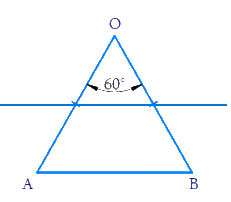\begin{align}\therefore \angle {{OAB}}\,= \,\angle {{OBA}} \end{align} (Angles opposite equal $$= \rm{}x^\circ$$ (say) sides in a triangle)

\begin{align}\angle {{AOB + }}\angle {{OAB + }}\angle {{OBA = 18}}{{{0}}^{{o}}}\end{align} (angle sum of triangle)

\begin{align}{{{60}^\circ } + x^\circ + x^\circ }&= {{{180}^\circ }}\\{2{{x^\circ}}} &= {{{180}^\circ } - {{60}^\circ }}\\x^\circ &= {\frac{{{{120}^\circ }}}{2}}\\x^\circ &= {{{60}^\circ }}\end{align}

\begin{align}&= \frac{\theta }{{{{360}^\circ }}} \times \pi {r^2}\\ &= \frac{{{{60}^\circ }}}{{{{360}^\circ }}} \times \pi {r^2}\end{align}

\begin{align} &= {\frac{{{{60}^\circ }}}{{{{360}^\circ }}} \times \pi {r^2} - \frac{{{r^2}\sqrt 3 }}{4}}\\& = {\frac{{\pi {r^2}}}{6} - \frac{{{r^2}\sqrt 3 }}{4}}\end{align}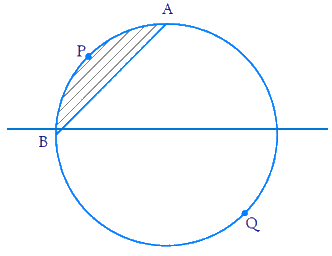\begin{align}= \pi {r^2} \end{align} - $$\text{Area of minor segment}$$

Steps: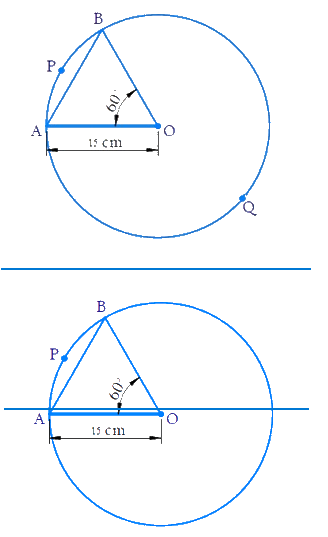Here,\begin{align}\rm{Radius, }r = 15\,cm\,\,\,\,\,\,\,\,{\rm{\theta }} = 60^\circ\end{align}

\begin{align}\text{Area of the sector OAPB} = \frac{{\rm{\theta }}}{{{{360}^{\rm{\circ}}}}} \times \pi {r^2}\end{align}

\begin{align}& = {\frac{{{{60}^\circ }}}{{{{360}^\circ }}} \times \pi {r^2}}\\&= { \frac{1}{6} \times 3.14 \times {{15}^2}}\\& = {117.75\,\rm{{c}}{{{m}}^2}}\end{align}

In $$\Delta \,{{OAB}},$$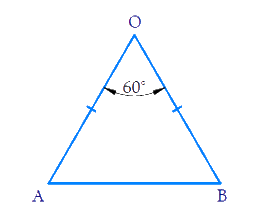\begin{align}OA = OB = r\,\,\,\,\,\,\,\,\,\,\,\,\,\,{\rm{}}\left( {{\text{radii of the circle}}} \right)\end{align}

\begin{align} \angle OBA=\angle OAB \end{align} ( Angles opposite equal sides in a triangle)

\begin{align} \angle {AOB+}\angle {OBA+}\angle {OAB}&=180^\circ \quad\left( \text{Angle sum of a triangle} \right) \\\angle {OBA+}\angle {OAB}&=18{{{0}}^{{o}}}{-60^\circ} \\ {2}\angle {OAB}&=120^{\circ} \\ \angle {OAB}&={{60}^{\circ }} \end{align}

$$\therefore \,\Delta {{OAB}}$$ is an equilateral triangle (as all its angles are equal)

$$\text{Area of }\Delta AOB$$\begin{align}&= \frac{{\sqrt 3 }}{2}{({{side}})^2}\\& = \frac{{\sqrt 3 }}{2}{r^2}\\& = \frac{{\sqrt 3 }}{4}{(15)^2}\\& = \frac{{225\sqrt 3 }}{4}\\ &= 56.25\sqrt 3 \\ &= 56.25 \times 1.73\\& = 97.3125\,\rm{{ cm}^2}\end{align}

Area of minor segment $$APB =$$ Area of sector $$OAPB\,-\,$$Area of $$\Delta AOB$$

\begin{align}&= 117.75 - 97.3125\\ &= 20.4375\,\rm{{c}}{{{m}}^2}\end{align}

Area of major segment $$AQB\,=\,$$ Area of circle $$-\,$$Area of minor segment $$APB$$

\begin{align}&= \pi {(15)^2} - 20.4375\\&= 3.14 \times 225 - 20.4375\\&= 706.5 - 20.4375\\&= 686.0625\,\rm{{ cm}}^2 \end{align}

Learn from the best math teachers and top your exams

• Live one on one classroom and doubt clearing
• Practice worksheets in and after class for conceptual clarity
• Personalized curriculum to keep up with school